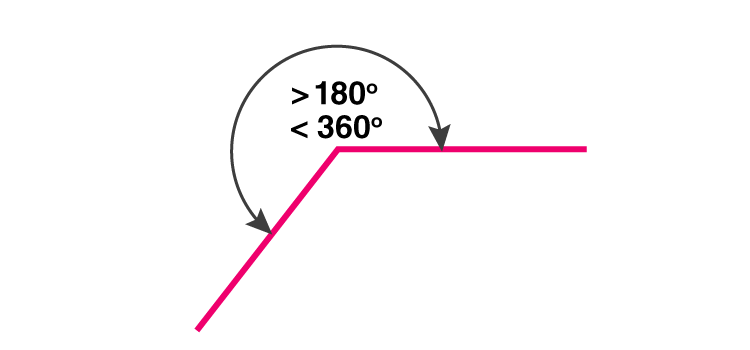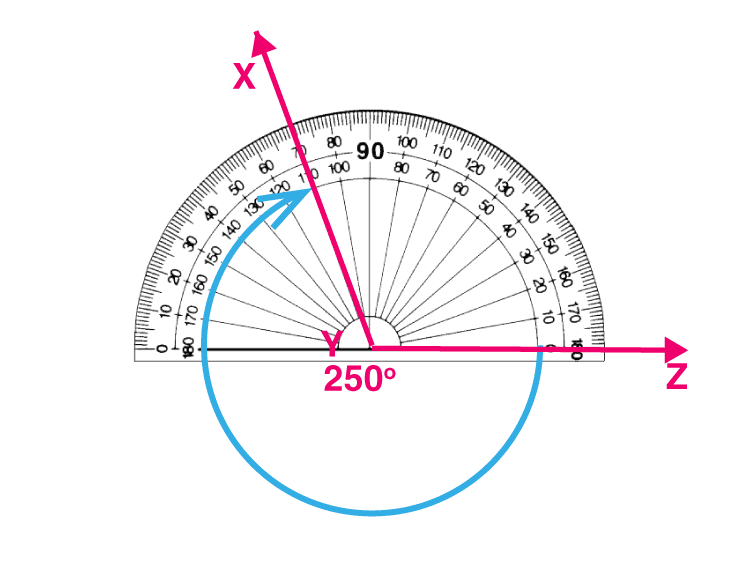# Reflex Angle

A reflex angle is an angle which is more than 180 degrees and less than 360 degrees. For example, 270 degrees is a reflex angle. In geometry, there are different types of angles such as acute, obtuse and right angle, which are under 180 degrees.

 Fact: For every acute and obtuse angle, there is a reflex angle. Suppose, x is an acute or obtuse angle, and r is the reflex angle, then: x+r = 360 r=360-x Therefore, if we know the value of acute or obtuse angle, we can easily find the related reflex angle.

An acute angle is the smallest angle measuring between 0 to 90 degrees, whereas the obtuse angle measures between 90 and 180 degrees. The right angle is exactly equal to 90 degrees.

Apart from these, there are three other types such as straight, reflex and full rotation. A straight angle is equal to 180 degrees and full rotation is equal to 360 degrees. Full rotation is also termed as a full circle. Let us learn here how an angle is said to be reflex.

 Angles Measure Acute Angle 0°

## Reflex Angle Definition

As we have already discussed in the introduction, the reflex angle is the angle greater than 180 degrees and less than 360 degrees. In the figure given below, the angle is a reflex angle which lies between 180° and 360°.A reflex angle is equal to the sum of 180 degrees and any of the primary angles (acute, right and obtuse angles). Therefore,

• Reflex angle = 180° + Acute angle
• Reflex angle = 180° + Right angle
• Reflex angle = 180° + Obtuse angle

## Degree

As per the definition of reflex angle, any degree which lies between straight angle (180°) and full rotation (360°) is a reflex angle. Hence, 181°, 190°, 200°, 210°, 220°, 230°, 240°, 250°, 260°, 270°, 280°, 290°, 300°, 310°, 320°, 330°, 340°, 350°, 359°, are all reflex angles.

## How to measure a reflex angle?

There are two ways to measure the reflex angle.

• By measuring the interior angle and subtracting it from 360 degrees.
• By using a protractor to measure the reflex angle itself

Steps to measure a reflex angle:

Since the measure of a reflex angle is greater than 180 degrees and the protractor has the maximum measure of 180 degrees, therefore we need to follow the below steps to measure the reflex angle.1. Place the center of the protractor above the center point where the two lines of the angle lie and make sure the line goes through 0°.
2. Now mark with the point where 180 degrees completes.
3. Now we need to measure the rest of the angle from the 180 degrees till the given second line.
4. After measuring the angle beyond 180 degrees, we need to add it to 180° to get the required reflex angle.
5. You can check the resultant angle, by measuring the interior angle and subtracting it from 360°.

### Worksheets

Draw a reflex angle for the given measurements:

• 190°
• 270°
• 370°
• 345°
• 241°

## Frequently Asked Questions – FAQs

### What is a reflex angle?

A reflex angle is an angle that measures more than 180 degrees and less than 360 degrees.

### What are the examples of reflex angle?

The examples of reflex angle are 190 degrees, 220 degrees, 270 degrees, 320 degrees, etc.

### What is a complete angle?

A complete angle is equal to 360 degrees. It is also called full rotation or full angle.

### Is 180 degrees a reflex angle?

180 degrees is not a reflex angle it is a straight angle.

### How to draw a reflex angle?

To draw a reflex angle, we can put the protractor upside-down, and mark an angle pointing downwards, which will be more than 270 degrees and less than 360 degrees.

### What is a zero angle?

An angle that measures zero degrees, is called zero angle.

### What are the other 5 types of angles apart from reflex angle?

Apart from reflex angle, the other 5 types of angles are:
Acute angle
Obtuse angle
Right angle
Straight angle
Full rotation

Test your Knowledge on Reflex Angle

#### 1 Comment

1. Sumanth koppula

Byjus is very helpful for learning